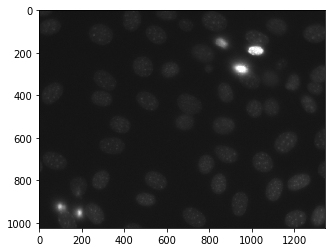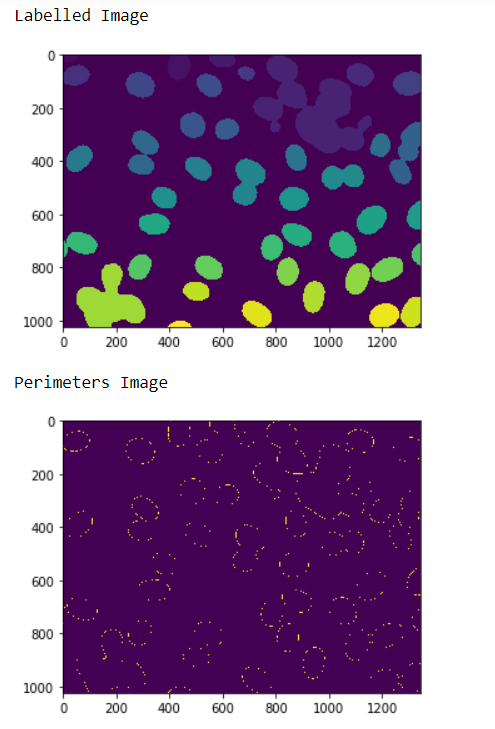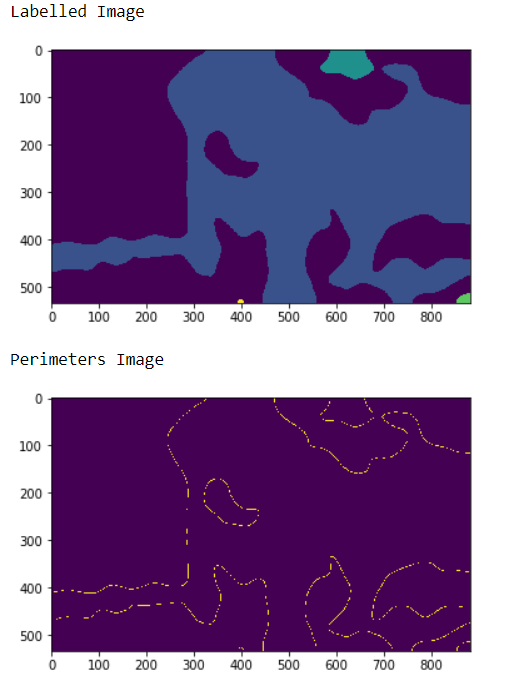# Mahotas – Perimeter of Objects in binary image

In this article we will see how we can get the perimeter of objects in binary images in mahotas. For this we are going to use the fluorescent microscopy image from a nuclear segmentation benchmark. We can get the image with the help of command given below

`mhotas.demos.nuclear_image()`

Below is the nuclear_imageA pixel is part of an object perimeter if its value is one and there is at least one zero-valued pixel in its neighborhood. By default the neighborhood of a pixel is 4 nearest pixels, but if we can set it to 8 then 8 nearest pixels will be considered.

In order to do this we will use `mahotas.labeled.bwperim` method

Syntax : mahotas.labeled.bwperim(image, n)

Argument : It takes numpy.ndarray object i.e labelled image preferred black and white and integer i.e nearest pixels which is optional

Return : It returns numpy.ndarray object i.e a boolean image

Note : The input of the this should should be the filtered image object which is labeled
In order to filter the image we will take the image object which is numpy.ndarray and filter it with the help of indexing, below is the command to do this

`iamge = image[:, :, 0]`

Example 1 :

 `# importing required libraries ` `import` `mahotas ` `import` `numpy as np ` `from` `pylab ``import` `imshow, show ` `import` `os ` ` `  `# loading nuclear image ` `f ``=` `mahotas.demos.load(``'nuclear'``) ` ` `  `# setting filter to the image ` `f ``=` `f[:, :, ``0``] ` ` `  `# setting gaussian filter ` `f ``=` `mahotas.gaussian_filter(f, ``4``) ` ` `  `# setting threshold value ` `f ``=` `(f> f.mean()) ` ` `  `# creating a labeled image ` `labeled, n_nucleus ``=` `mahotas.label(f) ` ` `  ` `  `# showing the labeleed image ` `print``(``"Labelled Image"``) ` `imshow(labeled) ` `show() ` ` `  `# getting perimeters ` `relabeled ``=` `mahotas.labeled.bwperim(labeled) ` ` `  ` `  `# showing the image ` `print``(``"Perimeters Image"``) ` `imshow(relabeled) ` `show() `

Output :Example 2 :

 `# importing required libraries ` `import` `numpy as np ` `import` `mahotas ` `from` `pylab ``import` `imshow, show ` `  `  `# loading iamge ` `img ``=` `mahotas.imread(``'dog_image.png'``) ` `    `  `# filtering the imagwe ` `img ``=` `img[:, :, ``0``] ` `     `  `# setting gaussian filter ` `gaussian ``=` `mahotas.gaussian_filter(img, ``15``) ` `  `  `# setting threshold value ` `gaussian ``=` `(gaussian > gaussian.mean()) ` `  `  `# creating a labeled image ` `labeled, n_nucleus ``=` `mahotas.label(gaussian) ` `   `  `print``(``"Labelled Image"``) ` `# showing the gaussian filter ` `imshow(labeled) ` `show() ` `  `  `# getting perimeters ` `relabeled ``=` `mahotas.labeled.bwperim(labeled, ``8``) ` ` `  ` `  `# showing the image ` `print``(``"Perimeters Image"``) ` `imshow(relabeled) ` `show() `

Output :My Personal Notes arrow_drop_upCheck out this Author's contributed articles.

If you like GeeksforGeeks and would like to contribute, you can also write an article using contribute.geeksforgeeks.org or mail your article to contribute@geeksforgeeks.org. See your article appearing on the GeeksforGeeks main page and help other Geeks.

Please Improve this article if you find anything incorrect by clicking on the "Improve Article" button below.

Article Tags :

Be the First to upvote.

Please write to us at contribute@geeksforgeeks.org to report any issue with the above content.